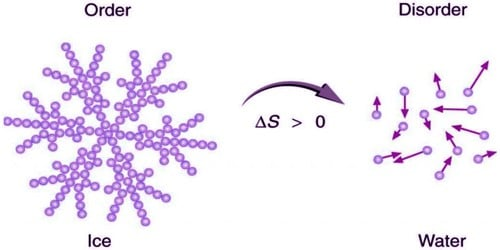# Explain Characteristics of Entropy ‘S’

Entropy is a determination of the level of uncertainty of the molecules comprising the structure. The entropy of the structure is precise in terms of the changes the structure has undergone from the preceding state to the last state. Entropy is the function of a measure of heat which shows the option of conversion of that heat into work. Thus the entropy is constantly measured as the transform in entropy of the system denoted by ∆S and not merely S.

Characteristics of entropy ‘S’

i) The term ‘S’ entropy is evolved from the formulation of II law of thermodynamics as a thermodynamic state function.

ii) Entropy change ‘ΔS’ of a system under a process is defined as the constant equal to the ratio of the heat change accompanying a process at constant temperature to the temperature of the system under process. The process should be reversible at that temperature.

∆Srev = ∆qrev/T (K)

Heat, q is not a state function, But for a reversible process Δq = (q2 –q1) divided by temperature (T) of the process is a state function.

iii) A spontaneous process is accompanied by an increase in the ‘disorder’ (or) ‘randomness’ of the molecules constituting the system. Entropy increases in all spontaneous processes. Hence entropy may be regarded as a measure of disorder (or) randomness of the molecules of the system.

iv) When a system undergoes a physical (or) a chemical process, there occurs a change in the entropy of the system and also in its surroundings. This total change in the entropy of the system and its surroundings is termed as the entropy change of the universe brought about by the process. For an isothermal process (T=constant), the entropy change of the universe during a reversible process is Zero.

The entropy of the universe increases in an irreversible process. It increases when the heat is supplied irrespective of the fact whether temperature changes or not.

v) The energy of the universe remains constant although the entropy of the universe tends to a maximum. Whether temperature changes or not the entropy decreases when the heat is rejected.

vi) For a spontaneous process, at constant T, ΔS is positive (ΔS > 0). ΔS is positive (ΔS > 0). For an equilibrium process, ΔS is zero. In all the adiabatic processes, the entropy remains stable.

For a non-spontaneous process, ΔS is negative or (ΔS < 0).

(vii) Higher the disorder, greater is the increase in entropy. The entropy of the system is not measured in absolute terms; rather it is measured in relative terms.

The increase in entropy is diminutive when the heat is added at a bigger temperature and is larger when the heat is added at a lower temperature. If at all it is essential to evaluate the value of the entropy at an exacting state of the system, then zero value of entropy is assigned to the earlier selected state of the system. Entropy is an extensive property i.e. it depends on the mass of the system not on the path by which the process takes place.